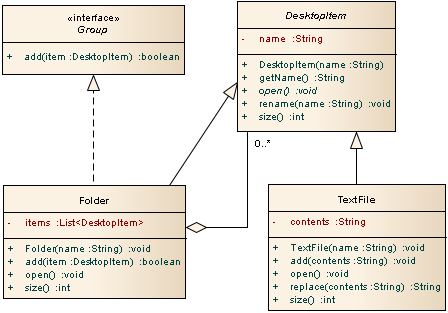# Class Activities

Please try to complete these before class on Tuesday. Feel free to work with others in your subgroup.

## Activity 1

Implement the abstract `Calculable` class below:

 Calculable +plus(that: Calculable): Calculable +minus(that: Calculable): Calculable +doubleValue(): double +intValue(): int

## Activity 2

Implement a concrete `Double` class that extends the `Calculable` class.

## Activity 3

Implement a concrete `Integer` class that extends the `Calculable` class.

## Activity 4

Modify the Complex class (see Putting it All Together section) so that the following is possible:

```Calculable c = new Complex(3, 2);
```

## Activity 5• Write the class declarations (first line) for all of the classes in the diagram above.
• List all of the abstract methods shown in the diagram.
• Implement the constructor for the `TextFile` class.
• Implement the constructor for the `Folder` class.
• Suppose that the size of a `Folder` object is defined as the size of all the objects contained in the folder (and subfolders) plus the number of characters representing the name of the folder. Implement the `size()` method for the `Folder` class.